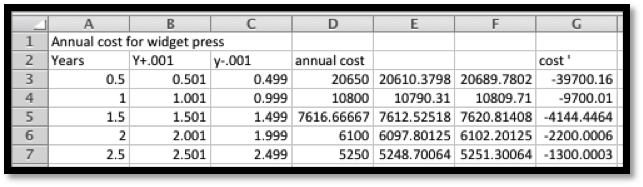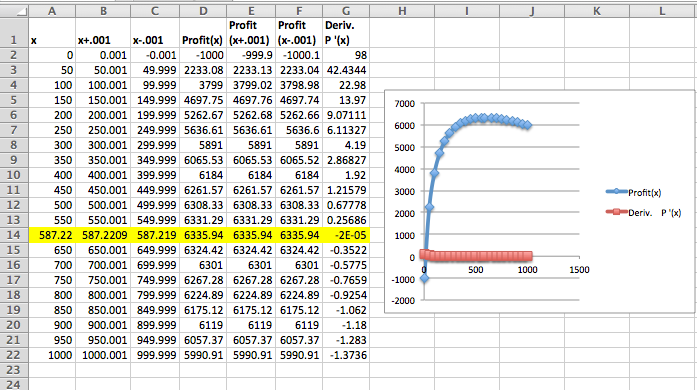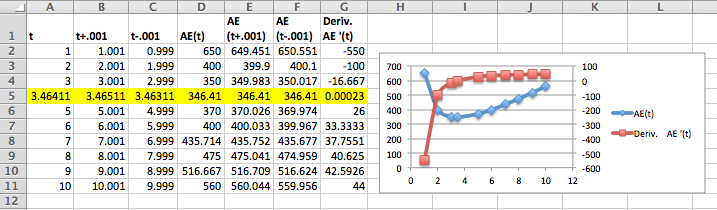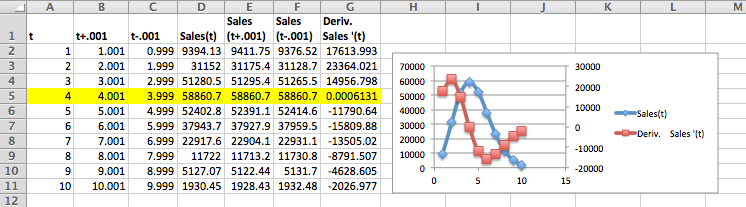## Section3.4Optimization

In Section 3.2 we noticed that the high and low points of the graph of a function often coincided with a point where the derivative of the function was 0. In a business setting, we are often concerned with finding the maximum and minimum values of a function, because one or the other will be a best, or optimum value. We typically want to maximize functions like profit, utility, revenue, and market share. We typically want to minimize functions like cost and liability. We will use the same basic process to optimize, whether the extremum we are finding is a maximum and minimum.
Recall, we said that the derivative can be thought of as the slope of the apparent line, obtained by zooming in on the graph of a function. Clearly, we cannot have an extremum at an interior point of the domain if the derivative is nonzero, because we could go either higher or lower by moving a little to the right or left. Thus we can only have extrema at a critical point, a place when the derivative is zero or undefined, or at an endpoint where we cannot go both left and right. This gives us a small list of candidate points for the optimum value.
Our process for optimization will be to find all the candidate points, then to see which gives the highest and lowest values. When our curve has a point that is a maximum or minimum in some interval around the point, we call it a relative maximum or minimum. If it is the highest or lowest point for the whole domain of the function it is called a global maximum or minimum.

### Example3.4.1.Profit function for widgets.

We have determined that the profit function for selling widgets is
\begin{equation*} \profit(\quantity)=\quantity*(400-\quantity)-5000\text{,} \end{equation*}
with the function valid on the interval $$0\le \quantity\le 500\text{.}$$ Find the minimum and maximum profit in the given interval.
Solution 1. Solution A: without calculus

### Example3.4.11.Minimizing expenses.

We are running a business and want to minimize equipment expenses. For a particular piece of equipment the costs can be broken into initial outlays to buy the equipment, fixed annual expenses to keep the equipment in the inventory, and repair costs which we anticipate rising as the equipment gets older. A widget press costs $10,000 to buy, and the operating expenses are$500 a year, and the total repair costs are $$300t^2$$ over the first $$t$$ years. What is the optimal length of time to use a widget press before replacing it?
Solution.
My annual cost equation is total cost divided by $$t\text{.}$$ This annual cost is:
\begin{equation*} \AnnualCost(t)=10000/t+500+300t\text{.} \end{equation*}
I create a spreadsheet that calculates the cost and its derivative over the first 10 years.#### 12.

The cost equation for gizmos is $$\cost(q)=1000+3q$$ and the demand function is $$\price(q)=500-3q\text{.}$$ Find the maximum profit.

#### 13.

The cost equation for gadgets is $$\cost(q)=1000+2q+.0001q^2$$ and the demand function is $$\price(q)=100/(1+.01q)\text{.}$$ Find the maximum profit.
Solution.
\begin{align*} \profit(q)\amp = \revenue(q)- \cost(q)\\ \amp =q*100/(1+.01q)- (1000+2q+.0001q^2)\text{.} \end{align*}
Assuming that the price should be a positive number does not help us in this problem. We can check, but all we get is that $$q \gt -100\text{,}$$ but $$q$$ should be positive.
If domain restrictions are not obvious or the algebra is challenging we can resort to trial and error. The small coefficients (.0001 for instance) do suggest we have fairly large values for $$q\text{.}$$#### 16.

Suppose your cost function is $$\cost(q)=10000(0.8)^{.1q}+.1q^2\text{.}$$ Find the quantity that minimizes price.

#### 17.

With rental property, a simplified model of costs spreads the purchase cost over the time that the property is held and assume that repair costs will rise the longer the property is held. This gives a formula for annual expenses as
\begin{equation*} \AnnualExpense(t)=(\Pprice)/t+\RepairFactor*t^r\text{,} \end{equation*}
where $$r$$ is a positive number that depends on the type of property. Assume for carpet cleaners that the replacement cost is $600, that the repair costs in the first year are$50, and $$r=1\text{.}$$ Find the length of time the property should be held to minimize the annual expense.
Solution.
In this instance the Annual Expense function is
\begin{equation*} \AnnualExpense(t)=600/t+50*t^1=600/t+50t\text{.} \end{equation*}
Note that the function is not defined when $$t = 0\text{.}$$ So we will graph the function and the derivative for $$1 \le t \le 10\text{.}$$ The property should be held for 3.5 years.#### 18.

With rental property, a better model factors in the depreciation of the property and how much can be recovered by selling the property used. If we use a 5 year straight line depreciation the formula becomes
\begin{equation*} \AnnualExpense(t)=(\Pprice*(1-0.2t))/t+\RepairFactor*t^r\text{.} \end{equation*}
Repeat the assumptions from the problem above. Assume for carpet cleaners that the replacement cost is $600, that the repair costs in the first year are$50, and $$r=1\text{.}$$ Find the length of time the property should be held to minimize the annual expense.

#### 19.

The annual sales rate for a new toy is found to be $$\Sales(t)=10000 t^2 \exp(-t^2/16)\text{.}$$ Find the month that maximizes sales.
Solution.
If we are minimizing according to month, we will graph the function for $$1 \le t \le 12\text{.}$$GoalSeek does not improve our estimate, and we see that the maximum sales must occur when $$t = 4\text{.}$$ The derivative is (close to) 0 there and this means our sales are maximized in April (assuming 1 represents January).

#### 20.

 Production level 1903 2424 3065 3424 4076 Profit $828,560.10$942,625.40 $1,006,167.50$987,980.40 \$929,780.40
mathstat.slu.edu/~may/ExcelCalculus/external/Examples/Section-3-4-Examples.xlsx